The dice

What is the probability of events that if we throw a dice is rolled less than 6?

Result

p =  0.833

Solution:Leave us a comment of example and its solution (i.e. if it is still somewhat unclear...):Be the first to comment!To solve this example are needed these knowledge from mathematics:

Would you like to compute count of combinations?

Next similar examples:

1. Sum 10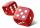What is the probability that two dice throw twice in a row will result the sum of 10?
2. Today in schoolThere are 9 girls and 11 boys in the class today. What is the probability that Suzan will go to the board today?
3. In the orchardIn the orchard, they planted 25 apple trees, 20 pears, 15 plums and 40 marbles. A strong late frost, however, destroyed a fifth of all new trees. Unfortunately, it was all the trees of one kind of fruit. What is the probability that the plums have died out
4. CardsSuppose that are three cards in the hats. One is red on both sides, one of which is black on both sides, and a third one side red and the second black. We are pulled out of a hat randomly one card and we see that one side of it is red. What is the probabi
5. Probability - tickets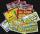What is the probability that when you have 25 tickets in 5000 that you wins the first (one) prize?
6. Win in raffle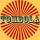The raffle tickets were sold 200, 5 of which were winning. What is the probability that Peter, who bought one ticket will win?
7. Seagull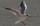Artificially created island in the shape of a circle with a radius of 50 m is overgrown with grass. The only exception is a landing area for helicopters in the shape of a rectangle measuring 15 m and 8 m. What is the probability that the flying seagull (wi
8. Components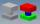In the box are 8 white, 4 blue and 2 red components. What is the probability that we pull one white, one blue and one red component without returning?
9. First manWhat is the likelihood of a random event where are five men and seven women first will leave the man?
10. Theorem proveWe want to prove the sentence: If the natural number n is divisible by six, then n is divisible by three. From what assumption we started?
11. CandiesIn the box are 12 candies that look the same. Three of them are filled with nougat, five by nuts, four by cream. At least how many candies must Ivan choose to satisfy itself that the selection of two with the same filling? ?
12. ShootersIn army regiment are six shooters. The first shooter target hit with a probability of 49%, next with 75%, 41%, 20%, 34%, 63%. Calculate the probability of target hit when shooting all at once.
13. AntennasIf you give me two antennas will be same. If you give me again your two antenna I have a 5× so many than you. How many antennas have both mans?
14. CandiesIf Alena give Lenka 3 candy will still have 1 more candy. If Lenka give Alena 1 candy Alena will hame twice more than Lenka. How many candies have each of them?In six baskets, the seller has fruit. In individual baskets, there are only apples or just pears with the following number of fruits: 5,6,12,14,23 and 29. "If I sell this basket," the salesman thinks, "then I will have just as many apples as a pear." WhichIf you earned 80% of the possible 40 points, how many points did you miss to get 100%?Find g(1) if g(x) = 3x - x2 Find f(5) if f(x) = x + 1/2